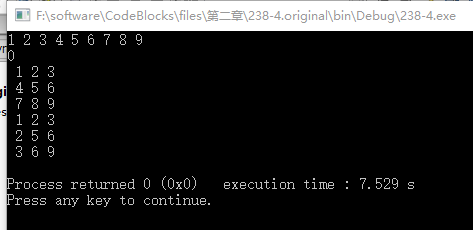#include
#include
#define N 3
#define M 3
/*238-4.编写一个函数（参数用指针）将一个3×3矩阵转置。*/
void matrixtranspose(int (*p)[M],int(*p1)[M])
{
int i,j;
for(i=0;i<N;i++)
{
for(j=0;j<M;j++)
{
((p1+j)+i)=*(*(p+i)+j);
}
}
}
void display(int (*p)[M])
{
int i,j;
for(i=0;i<N;i++)
{
for(j=0;j<M;j++)
{
printf("%2d",*(*(p+i)+j));
}
printf("\n");
}
}
int main()
{
int i,j,(*p)[M],a[N][M]={0},(*p1)[M];
for(i=0;i<N;i++)
{
for(j=0;j<M;j++)
{
scanf("%d ",&a[i][j]);
}
}
p=a;
display(p);
p1=a;
matrixtranspose(p,p1);
display(p1);
return 0;
}``````
``````

2个回答

//p1和p指向同一个指针a 所以转置交换数据时出错 重新定义b数组 p1指向b即可
int main()
{
int i, j, (*p)[M], a[N][M] = { 0 }, b[N][M] = { 0 },(*p1)[M];
for (i = 0; i<N; i++)
{
for (j = 0; j<M; j++)
{
scanf_s("%d ", &a[i][j]);
}
}
p = a;
display(p);
p1 = b;
matrixtranspose(p, p1);
display(p1);
return 0;
}/*在主函数中定义整型数组a，从键盘输入值赋给数组元素后，调用zhuanz函数将a数组转置，并输出转置后的数组。*/ #include<stdio.h> #include<stdlib.h> main() {/************found************/ } zhuanz(int (*p)) {/************found************/ }/*萌新崩溃，还有就是*（p)和*p什么区别，，*/

C语言：运用指针，输出3*3矩阵的转置阵。大佬们帮忙看看哪里出问题了

``` #include "stdafx.h" #include <stdio.h> int _tmain(int argc, _TCHAR* argv[]) { void fun(int *x); int a,i; int *p=&a; printf("请输入一个整形矩阵:"); for(i=0;i<3;i++) { scanf("%d %d %d",&a[i],&a[i],&a[i]); } fun(p); printf("输出转置矩阵：\n"); for(i=0;i<3;i++) { printf("%d %d %d\n",a[i],a[i],a[i]); } return 0; } void fun(int *x) { int m,n,s; for(m=0;m<3;m++) { for(n=0;n<3;n++) { s=*(x+3*m+n); *(x+3*m+n)=*(x+3*n+m); *(x+3*n+m)=s; } } } ``` 输入：1 2 3 4 5 6 7 8 9 输出：1 2 3 4 5 6 7 8 9 结果依然是原矩阵，是哪一步出问题了呢？

【C语言】有关数组转置与指针应用的题目，求大佬解答

#define M 4 #include <stdio.h> int main() { int a[M][M]={{1,2,3,4},{5,6,7,8},{9,10,11,12},{13,14,15,16}}; int i,j; for(i=0;i<M;i++) { for(j=0;j<M;j++) printf("%d\t", a[i][j]); printf("\n"); } for(i=0;i<M;i++) { for(j=0;j<M;j++) { int t=a[i][j]; a[i][j]=a[j][i]; a[j][i]=t; } } for(i=0;i<M;i++) { for(j=0;j<M;j++) printf("%d\t", a[i][j]); printf("\n"); } return 0; }

c语言简单题，求矩阵的转置矩阵

![图片说明](https://img-ask.csdn.net/upload/201901/04/1546611499_210346.png) 我的答案： ``` #include <stdio.h> #define N 10 void Transpose(int (*a)[N], int n); void Swap(int *x, int *y); void InputMatrix(int (*a)[N], int n); void PrintMatrix(int (*a)[N], int n); void InputMatrix(int (*a)[N], int n) { int i,j; for(i = 0;i < n ;i++) { for(j = 0;j < n; j++) { scanf("%d",&a[i*n + j]); } } } void Transpose(int (*a)[N], int n) { int i,j; for(i = 0;i < n ;i++) { for(j = 0;j < n; j++) { Swap(&a[i*n + j], &a[j*n + i]); } } } void Swap(int *x, int *y) { int temp; temp = *x; *x = *y; *y = temp; } PrintMatrix(int (*a)[N], int n) { int i,j; for(i = 0;i < n ;i++) { for(j = 0;j < n ; j++) { printf("%d\t", *a[i*n + j]); } printf("\n"); } } int main() { int n,s[N][N]; printf("Input n:"); scanf("%d",&n); printf("Input %d*%d matrix:\n",n,n); InputMatrix( s, n); Transpose( s, n); printf("The transposed matrix is:\n"); PrintMatrix( s, n); return 0; } ``` 请问哪里错了？该怎么改？

![图片说明](https://img-ask.csdn.net/upload/202004/08/1586314740_244236.png) 编写一个矩阵转置函数，矩阵的行数和列数在程序中由用户输入 ``` #include <iostream> using namespace std; /* run this program using the console pauser or add your own getch, system("pause") or input loop */ void matrixTranspose(int (*a)[cols],int rows,int cols) //矩阵转置函数 /*二维数组作为函数参数必须将列数写入形式参数中， 二维数组的作为函数参数的传入方法： 1.int sum_arr(int a[N][N],int size)size的值是行数，二维数组可以看作是 元素为数组的一维数组 example:C++.P207 2.int sum_arr(int (*a)[cols],int size) 两种方法函数中调用都要用a[i][j] 数组的长度也要作为参数传入，不在函数的内部定义大小*/ { int b[N][N]={0}; int i=0,j=0; for(i=0;i<N;i++) { for(j=0;j<N;j++) { b[j][i]=a[i][j]; } } for(i=0;i<N;i++) { for(j=0;j<N;j++) { a[i][j]=b[i][j]; } } cout<<"转置后的矩阵"<<endl; for(i=0;i<rows;i++) { for(j=0;j<cols;j++) { cout<<a[i][j]<<" "; } cout<<endl; } } int main(int argc, char** argv) { int rows=0,cols=0; int i=0,j=0; cout<<"请输入行数和列数"<<endl; cin>>rows>>cols; int a[rows][cols]={0}; cout<<"请输入矩阵元素"<<endl; for(i=0;i<rows;i++) { for(j=0;j<cols;j++) { cin>>a[i][j]; cout<<a[i][j]<<" "; } cout<<endl; } matrixTranspose(a,rows,cols); return 0; } ```

C语言调用函数实现矩阵自乘

（c语言）编写一个函数,由实参传来一个矩阵 A[M][N],按列输出它每一列上最大值和最小值 的差

``` #include<stdio.h> int main() {void judge(int a,int m,int n); int m,n; printf("请输入矩阵的行数和列数：\n"); scanf("%d%d",&m,&n); int a[m][n],i,j; printf("enter the matrix:\n"); for(i=0;i<m;i++) for(j=0;j<n;j++) scanf("%d",&a[i][j]); judge(a,m,n); return 0; } void judge(int a,int m,int n) {int max,min,i,j; for(j=0;j<n;j++) {max=a[j];min=a[j]; for(i=1;i<m;i++) {if(max<a[i][j]) max=a[i][j]; if(min>a[i][j]) min=a[i][j]; } printf("第%d列最大值和最小值的差是%d\n",j+1,max-min); } } ``` ![图片说明](https://img-ask.csdn.net/upload/201904/26/1556284345_790043.png) 为什么不对，求大佬解答？

1.编写并测试3×3 矩阵转置函数， 使用数组保存3×3 矩阵。 2.使用动态内存分配生成动态数组来重新完成上题（ n 阶方阵），使用指针实现函数的功能。 ``` #include<iostream> using namespace std; void change(int(*p1)); int main() { int(*p); p = new int; cout << "请输入3x3矩阵的9个元素：" << endl; for (int i = 0; i < 3; i++) { for (int j = 0; j < 3; j++) { cin >> *(*(p+i)+j); } } change(p); cout << "转置后矩阵为：" << endl; for (int i = 0; i < 3; i++) { for (int j = 0; j < 3; j++) { cout << *(*(p + i) + j) << " "; } cout << endl; } for (int i = 0; i < 3; i++) delete[] p[i]; delete[]p; return 0; } void change(int(*p1)) { for (int i = 0; i < 3; i++) { for (int j = 0; j < 3; j++) { if (j > i) { int t = *(*(p1 + i) + j); *(*(p1 + i) + j) = *(*(p1 + j) + i); *(*(p1 + j) + i) = t; } } } } ``` 用vs编译时出现了 > warning C4154: 删除数组表达式；转换到提供的指针 运行时出现 ![图片说明](https://img-ask.csdn.net/upload/202004/22/1587568081_180167.jpg) 我发现问题是出在 > for (int i = 0; i < 3; i++) > delete[] p[i]; 因为注释这两行后问题就都没了…… 有无更好的解决办法？

#include <stdio.h> #include <stdlib.h> #define N 3 #define M 3 /*238-4.编写一个函数（参数用指针）将一个3×3矩阵转置。*/ /*与题目无关代码还没写完的*/ void display(int (*p)[M]) { int i,j; for(i=0;i<N;i++) { for(j=0;j<M;j++) { printf("%2d",*(*(p+i)+j)); } printf("\n"); } } int main() { int i,j,(*p)[M],a[N][M]={0}; for(i=0;i<N;i++) { for(j=0;j<M;j++) { scanf("%d ",&a[i][j]); } } p=a; display(p); return 0; } ![图片说明](https://img-ask.csdn.net/upload/201912/01/1575186576_993448.png) ``` ```

Python代码实现飞机大战

Python数据分析与挖掘

92讲视频课+16大项目实战+源码+￥800元课程礼包+讲师社群1V1答疑+社群闭门分享会=99元 &nbsp; 为什么学习数据分析？ &nbsp; &nbsp; &nbsp; 人工智能、大数据时代有什么技能是可以运用在各种行业的？数据分析就是。 &nbsp; &nbsp; &nbsp; 从海量数据中获得别人看不见的信息，创业者可以通过数据分析来优化产品，营销人员可以通过数据分析改进营销策略，产品经理可以通过数据分析洞察用户习惯，金融从业者可以通过数据分析规避投资风险，程序员可以通过数据分析进一步挖掘出数据价值，它和编程一样，本质上也是一个工具，通过数据来对现实事物进行分析和识别的能力。不管你从事什么行业，掌握了数据分析能力，往往在其岗位上更有竞争力。 &nbsp;&nbsp; 本课程共包含五大模块： 一、先导篇： 通过分析数据分析师的一天，让学员了解全面了解成为一个数据分析师的所有必修功法，对数据分析师不在迷惑。 &nbsp; 二、基础篇： 围绕Python基础语法介绍、数据预处理、数据可视化以及数据分析与挖掘......这些核心技能模块展开，帮助你快速而全面的掌握和了解成为一个数据分析师的所有必修功法。 &nbsp; 三、数据采集篇： 通过网络爬虫实战解决数据分析的必经之路：数据从何来的问题，讲解常见的爬虫套路并利用三大实战帮助学员扎实数据采集能力，避免没有数据可分析的尴尬。 &nbsp; 四、分析工具篇： 讲解数据分析避不开的科学计算库Numpy、数据分析工具Pandas及常见可视化工具Matplotlib。 &nbsp; 五、算法篇： 算法是数据分析的精华，课程精选10大算法，包括分类、聚类、预测3大类型，每个算法都从原理和案例两个角度学习，让你不仅能用起来，了解原理，还能知道为什么这么做。

MFC一站式终极全套课程包

C++语言基础视频教程

C++语言基础视频培训课程：本课与主讲者在大学开出的程序设计课程直接对接，准确把握知识点，注重教学视频与实践体系的结合，帮助初学者有效学习。本教程详细介绍C++语言中的封装、数据隐藏、继承、多态的实现等入门知识；主要包括类的声明、对象定义、构造函数和析构函数、运算符重载、继承和派生、多态性实现等。 课程需要有C语言程序设计的基础（可以利用本人开出的《C语言与程序设计》系列课学习）。学习者能够通过实践的方式，学会利用C++语言解决问题，具备进一步学习利用C++开发应用程序的基础。

Python界面版学生管理系统

Tomcat服务器下载、安装、配置环境变量教程(超详细)

2019数学建模A题高压油管的压力控制 省一论文即代码

2019数学建模A题高压油管的压力控制省一完整论文即详细C++和Matlab代码，希望对同学们有所帮助

linux下利用/proc进行进程树的打印

2019 AI开发者大会

2019 AI开发者大会(AI ProCon 2019)是由中国IT社区CSDN主办的AI技术与产业年度盛会。多年经验淬炼，如今蓄势待发：2019年9月6-7日，大会将有近百位中美顶尖AI专家、知名企业代表以及千余名AI开发者齐聚北京，进行技术解读和产业论证。我们不空谈口号，只谈技术，诚挚邀请AI业内人士一起共铸人工智能新篇章！

Python数据分析师-实战系列

YOLOv3目标检测实战系列课程

《YOLOv3目标检测实战系列课程》旨在帮助大家掌握YOLOv3目标检测的训练、原理、源码与网络模型改进方法。 本课程的YOLOv3使用原作darknet（c语言编写），在Ubuntu系统上做项目演示。 本系列课程包括三门课： (1)《YOLOv3目标检测实战：训练自己的数据集》 包括：安装darknet、给自己的数据集打标签、整理自己的数据集、修改配置文件、训练自己的数据集、测试训练出的网络模型、性能统计(mAP计算和画出PR曲线)和先验框聚类。 (2)《YOLOv3目标检测：原理与源码解析》讲解YOLOv1、YOLOv2、YOLOv3的原理、程序流程并解析各层的源码。 (3)《YOLOv3目标检测：网络模型改进方法》讲解YOLOv3的改进方法，包括改进1：不显示指定类别目标的方法 （增加功能） ；改进2：合并BN层到卷积层 （加快推理速度） ； 改进3：使用GIoU指标和损失函数 （提高检测精度） ；改进4：tiny YOLOv3 （简化网络模型）并介绍 AlexeyAB/darknet项目。

2021考研数学张宇基础30讲.pdf

DirectX修复工具V4.0增强版

DirectX修复工具(DirectX Repair)是一款系统级工具软件，简便易用。本程序为绿色版，无需安装，可直接运行。 本程序的主要功能是检测当前系统的DirectX状态，如果发现异常则进行修复

Vue.js 2.0之全家桶系列视频课程

c语言项目开发实例

2019全国大学生数学建模竞赛C题原版优秀论文

2019全国大学生数学建模竞赛C题原版优秀论文，PDF原版论文，不是图片合成的，是可编辑的文字版。共三篇。 C044.pdf C137.pdf C308.pdf

Git 实用技巧

linux“开发工具三剑客”速成攻略

MySQL数据库面试题（2020最新版）